Next: Pipe, Sudden Enlargement Up: Fluid Section Types: Liquids Previous: Pipe, Manning   Contents

### Pipe, White-Colebrook

This is a straight pipe with constant section and head losses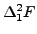defined by the formula: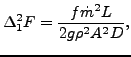(125)

where f is the White-Colebrook coefficient (dimensionless),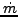is the mass flux, L is the length of the pipe, g is the gravity acceleration (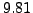m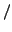s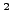), A is the cross section of the pipe and D is the diameter. The White-Colebrook coefficient satisfies the following implicit equation: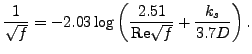(126)

Here,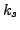is the diameter of the material grains at the surface of the pipe and Re is the Reynolds number defined by

 Re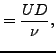(127)

where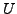is the liquid velocity and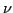is the kinematic viscosity. It satisfies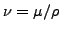where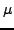is the dynamic viscosity.

The following constants have to be specified on the line beneath the *FLUID SECTION, TYPE=PIPE WHITE-COLEBROOK card:

• area of the cross section
• hydraulic diameter of the cross section (4 times the area divided by the perimeter)
• length of the pipe element; if this number is nonpositive the length is calculated from the coordinates of the pipe's end nodes.
• the grain diameter• form factor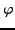of the cross section

The gravity acceleration must be specified by a gravity type *DLOAD card defined for the elements at stake. The material characteristicsandcan be defined by a *DENSITY and *FLUID CONSTANTS card. Typical values forare 0.25 mm for cast iron, 0.1 mm for welded steel, 1.2 mm for concrete, 0.006 mm for copper and 0.003 mm for glass.

The form factoris only used to modify the friction expression for non-circular cross sections in the laminar regime as follows: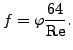(128)

Values forfor several cross sections can be found in . For a square cross section its value is 0.88, for a rectangle with a height to width ratio of 2 its value is 0.97.

By specifying the addition FLEXIBLE in the type label the user can create a flexible pipe. In that case the user specifies two nodes, the distance between them being the radius of the pipe. These nodes have to be genuine structural nodes and should not belong to the fluid network. The distance is calculated from the location of the nodes at the start of the calculation modified by any displacements affecting the nodes. Consequently, the use of the *COUPLED TEMPERATURE-DISPLACEMENT keyword allows for a coupling of the deformation of the pipe wall with the flow in the pipe. The following constants have to be specified on the line beneath the *FLUID SECTION, TYPE=PIPE WHITE-COLEBROOK FLEXIBLE card:

• node number 1
• node number 2
• length of the pipe element; if this number is nonpositive the length is calculated from the coordinates of the pipe's end nodes.
• the grain diameter• form factorof the cross section

Example files: pipe2.Next: Pipe, Sudden Enlargement Up: Fluid Section Types: Liquids Previous: Pipe, Manning   Contents
guido dhondt 2018-12-15